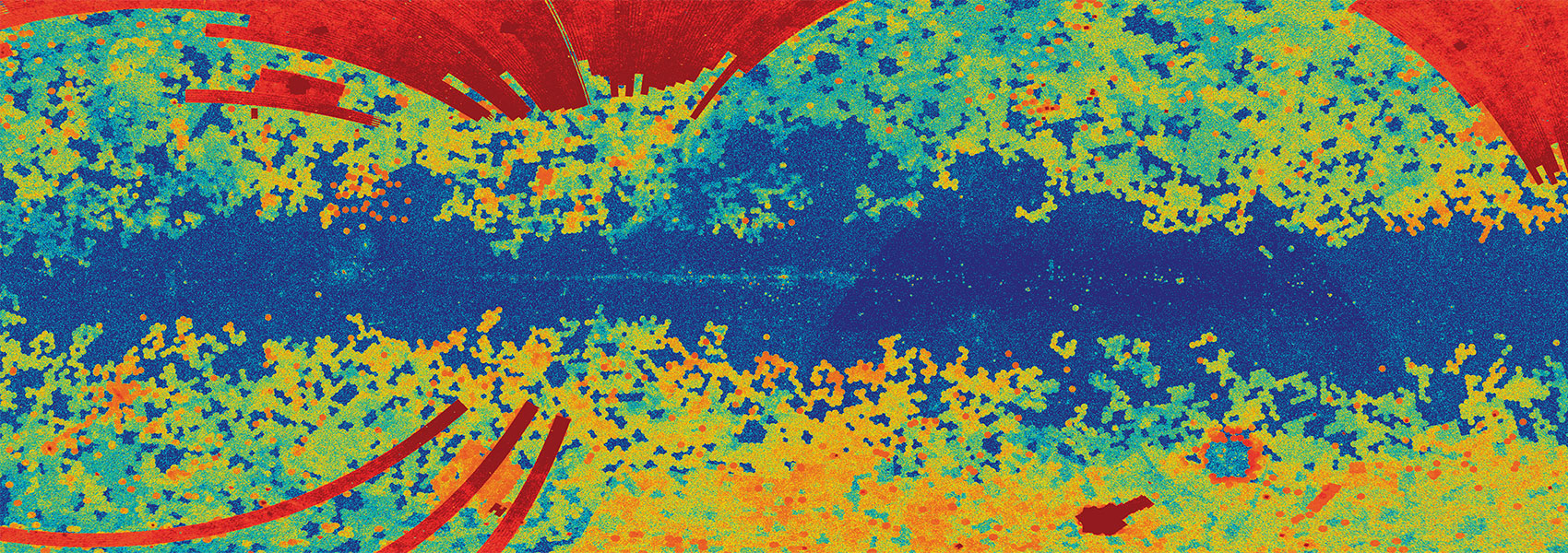# Calibrating the Planck Cluster Mass Scale with Cluster Velocity Dispersions

August 2017 • 2017ApJ...844..101A

Authors • Amodeo, Stefania • Mei, Simona • Stanford, Spencer A. • Bartlett, James G. • Melin, Jean-Baptiste • Lawrence, Charles R. • Chary, Ranga-Ram • Shim, Hyunjin • Marleau, Francine • Stern, Daniel

Abstract • We measure the Planck cluster mass bias using dynamical mass measurements based on velocity dispersions of a subsample of 17 Planck-detected clusters. The velocity dispersions were calculated using redshifts determined from spectra that were obtained at the Gemini observatory with the GMOS multi-object spectrograph. We correct our estimates for effects due to finite aperture, Eddington bias, and correlated scatter between velocity dispersion and the Planck mass proxy. The result for the mass bias parameter, (1-b), depends on the value of the galaxy velocity bias, {b}{{v}}, adopted from simulations: (1-b)=(0.51+/- 0.09){b}{{v}}3. Using a velocity bias of {b}{{v}}=1.08 from Munari et al., we obtain (1-b)=0.64+/- 0.11, I.e., an error of 17% on the mass bias measurement with 17 clusters. This mass bias value is consistent with most previous weak-lensing determinations. It lies within 1σ of the value that is needed to reconcile the Planck cluster counts with the Planck primary cosmic microwave background constraints. We emphasize that uncertainty in the velocity bias severely hampers the precision of the measurements of the mass bias using velocity dispersions. On the other hand, when we fix the Planck mass bias using the constraints from Penna-Lima et al., based on weak-lensing measurements, we obtain a positive velocity bias of {b}{{v}}≳ 0.9 at 3σ .